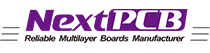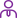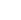### New Life with Your NEXTPCB!

Turnkey PCB manufacturing & assembly services.

LanguageLogin|Sign up
Blog >> Blog Details Page
The difference between Zero-ohm resistors, inductance, and single point grounding of magnetic beads
Posted:06:23 PM April 30, 2020 writer: NextPCB

Key introduction: The analog ground and single point grounding of digital ground.

All the ground will get together and go into the earth. It is called “floating ground” if the grounds are not connected, which will cause a pressure difference and accumulation of charge, resulting in static electricity. The ground is referenced zero potential, all voltages are obtained from the reference ground. The standard of the ground needs to be consistent, and all sorts of ground should be connected directly.

It is said that the ground will absorb all the charges and remain stable at all times; thus, it is the final ground reference point. Although some boards are not ungrounded, the power plant is grounded. The power on the board will eventually return to the power plant and back to the ground. The analog ground and digital ground will interfere with each other if they are directly connected.

It is not appropriate if the grounds are not connected directly. There are four ways to solve this problem:

① using magnetic beads to connect

② using capacitance to connect

③ using inductance to connect

④ using zero-ohm resistors to connect

The differences:

① The equivalent circuit of a magnetic bead is equivalent to a band-stop filter, which can only much suppress the noise of a certain frequency point. It is necessary to estimate the noise frequency in advance to select the appropriate model. The situation of uncertain or unpredictable frequency means the magnetic beads are not fit.

② The capacitor isolates DC but passes through AC, causing a floating ground.

③ The inductance is not stable because of the large volume and many stray parameters.

④ The Zero-ohm resistor is equivalent to a narrow current path, which can effectively limit the loop current and suppress noise. The resistor will decay across all frequency bands, and the zero-ohm resistor also has an impedance. This is better than than the magnetic bead.

Other uses of a zero-ohm resistor:

① The zero-ohm resistor on the PCB is only used for the reasons such as ease of debugging or compatible design, and doesn’t have any other functions.

② The zero-ohm resistor can be used as a jumper. It is necessary to paste the resistor if one section of the circuit is not in use.

③ When the matching circuit parameters are uncertain, the zero-ohm resistor can be used to replace related elements. When the parameters are determined during actual debugging, then using an element with a specific value to replace the zero-ohm resistor.

④ When measuring the current consumption of a part of the circuit, you can remove the zero-ohm resistor and connect the ammeter to measure the current consumption.

⑤ You can add a zero-ohm resistor when a layout is complicated at some part of the circuits.

⑥ In the case of high-frequency signals, zero-ohm resistor acts as an inductor or capacitor (it is related to external circuit characteristics), which is mainly to solve EMC problems between ground and ground or the power and IC Pin.

⑦ Single point grounding (it means protective grounding, working grounding, DC grounding is separated from each other on the equipment and becomes a separate system).

⑧ Fuse role

⑨ It is used in a current loop when bonding.

The shortest backflow path of the signal is broken when the split ground plane is divided, causing the signal circuit must detour and form a large loop area. Then the influence of the electric field and magnetic field becomes potent, which will easy to interfering/be interfered with. Bonding a zero-ohm resistor over the split area can provide a short loop path and reduce interferences.

⑩ Configuration circuits

Generally, no jumper and dial-code switch on the products are the best. Sometimes users will change the settings, resulting in misunderstandings. Using a zero-ohm resistor instead of a jumper to wield on the board can reduce the maintenance cost. Vacant jumpers are the same as antennas under high frequencies, then using a patch resistor will perform well.

⑪ Other uses Zero-ohm resistors can be used as a bonding when layout and used to debug/test, or a temporary replacement for other SMD devices. As temperature compensation devices, zero-ohm resistors are more often used for EMC countermeasures.

Besides, a zero-ohm resistance is smaller than the parasitic inductance of vias, and vias also affect the ground plane (because vias need to be dug). Also, different sizes of zero-ohm resistors allow different currents to pass through. the current usually is 1A for 0603 and 2A for 0805. Therefore, different zero-ohm resistors will be selected for different currents.

Packages are made according to the sizes of the magnetic beads and inductance when reserving positions for magnetic beads and inductances, which cause different sizes of zero-ohm resistors such as 0603 and 0805.

Attachment: the uses of 1 Ohm resistors

1 Ohm resistors are usually used to test in the circuits. For example, we can string a 1 Ohm resistor in a circuit when we need to measure the current in the circuit. The voltage measured at both ends is the current of the circuit. (I= U/R, then the measured voltage is the current value because the value of R is 1.)

Magnetic beads are specially used to suppress the high-frequency noise and peak interference on the signal wire and power wire.

Magnetic beads can be used to absorb ultra-high frequency(UHF) signals, like some RF circuits, PLLs, oscillation circuits, the circuits containing UHF memorizers (DDR, SDRAM, RAMBUS). These all need to add magnetic beads in the power input part. And the inductance is a kind of energy storage component, used in LC oscillation circuits, low and medium frequency filter circuits. Its application frequency range rarely exceeds 50MHZ. Magnetic beads have high resistivity and permeability, which is equivalent to the series circuits of resistance and inductance. But both the resistance and inductance vary with frequency.

Magnetic beads are mainly used to eliminate RF noise existing in the transmission line structure. Radiofrequency (RF) energy is an AC sine wave component superimposed on the DC transmission level, and the DC component is the required useful signal. But the RF energy is a useless electromagnetic interference traveling along the circuit and radiating (EMI).

Chips beads act as high-frequency resistors (attenuators) to eliminate these unwanted signal energies. The device allows the DC signal to pass through while filter AC signals and the high-frequency signals usually are higher than 30MHZ. However, low-frequency signals also will be affected by magnetic chip beads.

Magnetic beads have high resistivity and permeability, which is equivalent to the series circuits of resistance and inductance. But both the resistance and inductance change with frequency. Magnetic beads have better high-frequency filter characteristics than ordinary inductors and exist resistance at high frequencies. Thus, magnetic beads can maintain high impedance in a wide range of frequencies to enhance the filter effect of the frequency modulation(FM).

As a power supply filter, an inductor can be used. The electronic symbol of the magnetic bead indicates the inductance, but we can tell from the model that it uses a magnetic bead. In terms of circuit function, magnetic beads and inductors have the same principle with different frequency characteristics.

Note: Please notice that the unit of the magnetic beads is Ohm, not Henry. Because the unit of the magnetic beads is named according to the impedance generated at one certain frequency, so the unit of impedance is also Ohm.

Inductors

Inductance is a property of a closed-loop. The magnetic field occurs in the coil when the current passes through the coil, and the magnetic field will generate an induced current to resist the current passing through the coil. The interaction between the current and the coil is called the inductance, and its unit is Henry (H).

The difference between the magnetic bead and inductance:

Inductors are storage elements, and magnetic beads are energy conversion (consumption) parts. Inductors are mostly used for power supply filter circuits; their emphasis is placed on the suppression of conductive interference. Magnetic beads are mostly used for signal circuits, mainly for EMI.

Magnetic beads are good at absorbing ultra-high frequency(UHF) signals, like some RF circuits, PLLs, oscillation circuits, the circuits containing UHF memorizers (DDR, SDRAM, RAMBUS). These circuits need to add magnetic beads in the power input part. An inductor is a kind of storage elements which is used in LC oscillation circuits, low and medium frequency filter circuits. Its application frequency range rarely exceeds 50MHZ.In terms of circuit function, magnetic beads and inductors have the same principle with different frequency characteristics.

Still, need help? Contact Us:  support@nextpcb.com
Need a PCB or PCBA quote? Quote now

643 0 0 1
• PCB
Portotype
• PCB
Assembly
• SMD
Stencil
 Dimensions: (mm) × Quantity: (pcs) 5 5 10 15 20 25 30 40 50 75 100 120 150 200 250 300 350 400 450 500 600 700 800 900 1000 1500 2000 2500 3000 3500 4000 4500 5000 5500 6000 6500 7000 7500 8000 9000 10000 Other Quantities:(quantity*length*width is greater than 10㎡) OK Layers: 1 2 4 6 8 10 12 Thickness: 0.6 mm 0.8 mm 1.0 mm 1.2 mm 1.6 mm 2.0 mm 2.5 mmQuote now